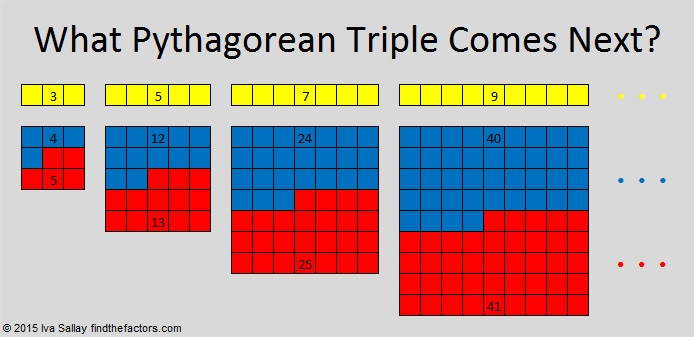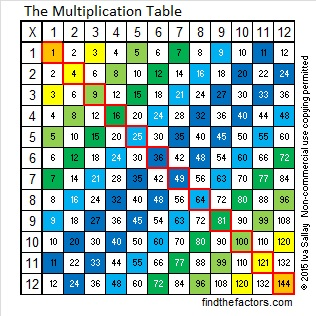# 371 What Pythagorean Triple Comes Next?

Could 371 be a prime number? Let’s do a quick prime number test on it.  2^371 (mod 371) = 340, not 2. Therefore, 371 is definitely a composite number. Scroll down to see its factoring information.If you can figure out the simple pattern that these odd Pythagorean triples make, you can predict the next one in the sequence FOREVER by squaring only one number, and without ever taking a single square root! Look at the blue and red squares in the graphic above.

• 4 + 5 = 9 = 3², and 3, 4, 5 make a Pythagorean triple.
• 12 + 13 = 25 = 5², and 5, 12, 13 is also a Pythagorean triple.
• 24 + 25 = 49 = 7², and 7, 24, 25 is another Pythagorean triple, and
• 40 + 41 = 81 = 9², and 9, 40, 41 is yet another Pythagorean triple!

What Pythagorean triple comes next? The answer is found by taking the next odd number, 11, squaring it to get 121 and dividing the 121 by 2. We round down to 60 for the 2nd number and round up to 61 for the 3rd number. Thus the next Pythagorean triple would be 11, 60, 61.

No matter how many times we repeat this pattern, we always get primitive Pythagorean triples. We can put all the triples in order to make a sequence that I call the Odd Primitive Pythagorean Triple Sequence:Every odd number is the short leg of at least one primitive Pythagorean triple! Here’s how I came to realize this amazing fact:

Last week I was thinking about the difference of two squares applied to integers in generalas I looked at this multiplication table.Everyone knows that the numbers in the boxes outlined in red are perfect squares, but most people do not realize that the numbers inside EVERY other colored square on this multiplication table can be expressed as the difference of two squares. The larger of those two squares will be the perfect square that is the same color.

For example, look at 5 x 11 or 55. If we count as we follow the light blue diagonal stripe from 55 to 64, we count 3 squares. That means that 55 = 64 – 3².

As I looked at the multiplication table I realized that some even numbered squares are colored and some are not, but EVERY odd number square is in color! That means that EVERY odd number on the table can be expressed as the difference of two squares in at least one way.

The light blue 9 from 1 x 9 is four squares away from the 25 that is outlined in red. 9 = 25 – 4². Since all those numbers are perfect squares, we can write 3² = 5² – 4² or the equivalent in Pythagorean Theorem form   3² + 4² = 5².

When an odd number is squared, the resulting perfect square is ALWAYS an odd number. Since all odd numbers can be written as the difference of two squares, The square of all odd numbers can be written as the difference of two squares. In other words, every odd number is a leg in a Pythagorean triple!

Let’s look at the factoring information for 371 and use it to find some Pythagorean triples.

• 371 is a composite number.
• Prime factorization: 371 = 7 x 53
• The exponents in the prime factorization are 1 and 1. Adding one to each and multiplying we get (1 + 1)(1 + 1) = 2 x 2 = 4. Therefore 371 has exactly 4 factors.
• Factors of 371: 1, 7, 53, 371
• Factor pairs: 371 = 1 x 371 or 7 x 53
• 371 has no square factors that allow its square root to be simplified. √371 ≈ 19.261371 is odd and has two odd prime factors. Here are some Pythagorean triples involving 371 as the short leg:

• 371, 68820, 68821 (This triple is a primitive from the odd primitive sequence.)
• 371, 1380, 1429 (A primitive, not from the odd primitive sequence. I’m also making a note that √(1380 + 1429) = 53 and √(1429 – 1380) = 7.)
• 7, 24, 25 multiplied by 53 becomes 371, 1272, 1325
• 53, 1404, 1405 multiplied by 7 becomes 371, 9828, 9835

371 is also the hypotenuse of a Pythagorean triple: 196, 315, 371 which is primitive 28, 45, 53 multiplied by 7.

## 2 thoughts on “371 What Pythagorean Triple Comes Next?”

1.wbhs62

More gorgeous, quirky stuff. Thank you!

•ivasallay

My pleasure!

This site uses Akismet to reduce spam. Learn how your comment data is processed.